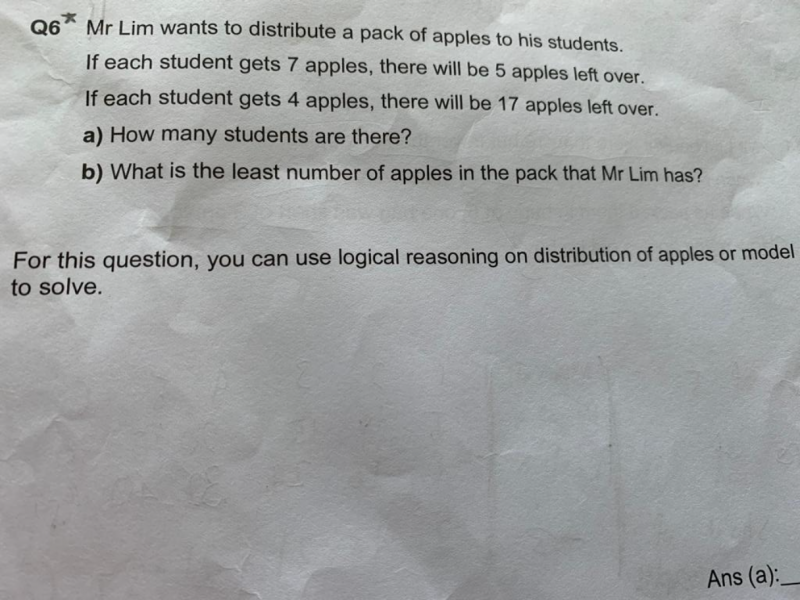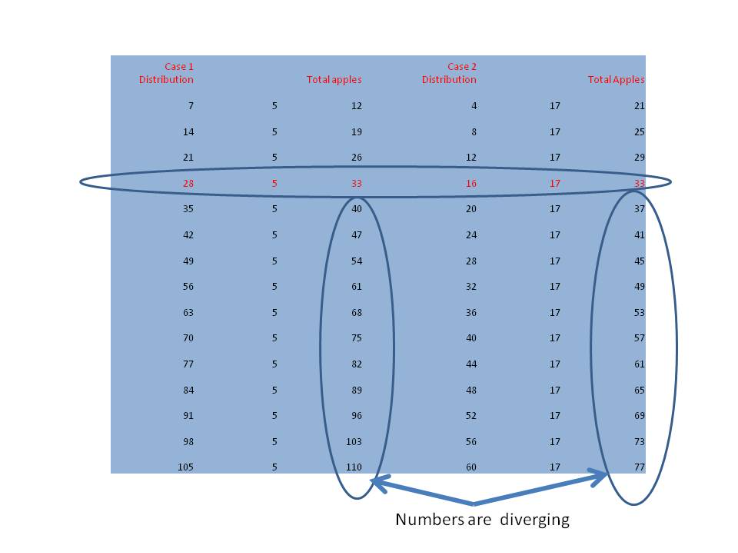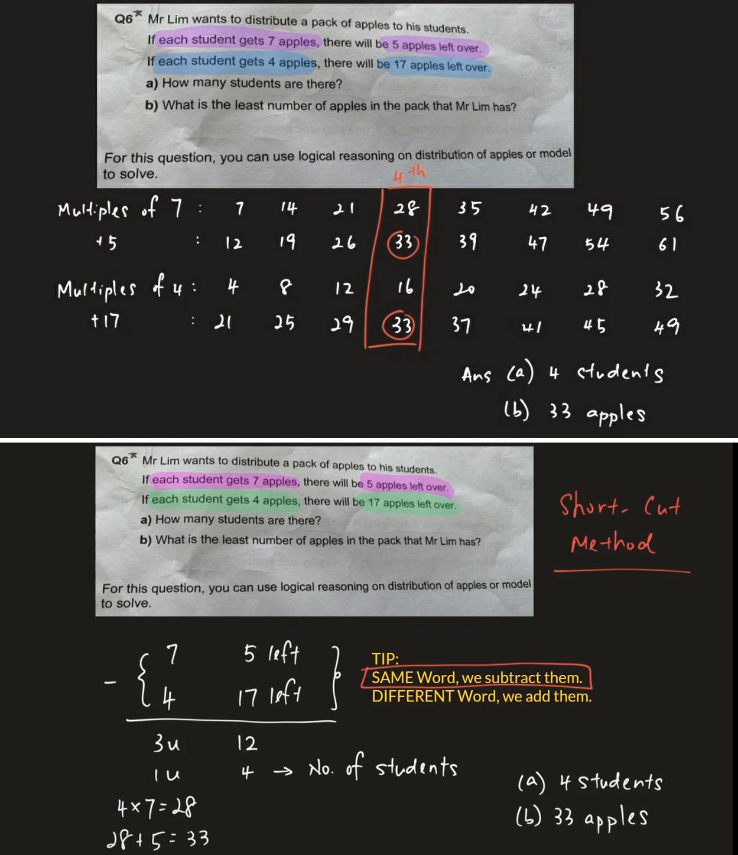# QuestionEither the question for part (b) is wrong or the answer is wrong.

The question should be:

(b) What is the number of apples in the pack that Mr Lim has?

The word least has to be deleted because there is ONE AND ONLY ONE ANSWER for this question which is 33 so “least” cannot be used in the question.

Only if there are multiple answers for a question, then least can be used, otherwise where is the comparison??   See the picture attached to see that the numbers for both cases will go in divergent ways, ie. there will NEVER be another answer that they will converge as EQUAL again.   Math concepts and language are VERY SPECIFIC, the question is grossly WRONG.

Now if the question is not wrong, then the answer should be 28 because 16 is too few to distribute case 1 and 33 is too many (as it is not the least) so it has to be 28 and it would not be linked to part (a)  due to the word LEAST.

I am however, inclined to think that the question (b) is set wrongly with the additional word ” least”  because the answer being 28 would be TOO DIFFICULT for a P4 to infer from the language as I have stated earlier.

Please feedback to the school if it is a school question.  If it is an assessment book question, please point out to the publisher.

0 Replies 2 Likes ✔Accepted Answer

```a) Let N be number of students. Then number of apples = 7N + 5 = 4N + 17.
So 3N = 17 - 5 = 12 and N = 4. There are 4 students.

b) Plug N = 4 to get number of apples = 7x4 + 5 = 33.
There is only ONE solution. So the least number of apples is 33.

Commentary:
a) The answer is the same as sushi88's and MyStudyRoom's alternate solution.
Nothing new there. It is a straight forward question after all.

However I feel that "List Out the Multiples" is not ALWAYS appropriate
for this TYPE of question. For instance, suppose the question says:
- If each student gets 7 apples, there are 5 left over
- If each student gets 4 apples, there are 29 left over

This means, 7N + 5 = 4N + 29. So N = (29-5)/(7-4) = 8 students.
If we do "List Out the Multiples", we get:```
 x 7 7 14 21 28 35 42 49 56 63 x 7 + 5 12 19 26 33 40 47 54 61 68 x 4 4 8 12 16 20 24 28 32 36 x 4 + 29 33 37 41 45 49 53 57 61 65
```There is a chance that the child will notice the repeated 33 first and
do not extend the list to 61 (which is the correct number of apples).
The child will then get quite confused as the number of students cannot
be worked out consistently.

Now, suppose the question was rephrased as:
- if the apples are packed into large boxes of 7, there are 5 left over
- if the apples are packed into small boxes of 4, there are 29 left over

In this case, the number of large boxes and small boxes may be DIFFERENT
and "List Out the Multiples" will be the correct method of solving this.
(We cannot solve the equation 7L + 5 = 4S + 29 uniquely.)

Also in this case, very importantly, it is legitimate to ask for the
LEAST number of apples.

b) Since there is exactly 1 solution, the answer to the LEAST number of
apples is technically the only one: 33. Same if they ask for the MOST. :-)

My gut feel is that this question was (somewhat mindlessly) modified from a
"large boxes/small boxes" type of question and that's why they are asking
for the least, even though there is only 1 solution.```

LOL!  You are right @mr.tan

The question is wrong in more than one way.

This question tries to do too much.  The moment how many students are there is being asked, it fixed the number of students there.   Students is not a variable.   So if student is not a variable, even if the next lot of apples equal at 61, students are 7 and 11 respectively.    In reality, can the number of students become 7 and 11?    So this option is not a permissible answer.

In view of that, there are no multiple possible solution for the apples except 33 if the question is phrased in the current way, hence the word “least” has to be deleted.

It is however good that you have explored the way the question could be phrased to get multiple possibilities and I agree with you that only if there is no unique solution, then “least” can be used to give only an answer for the purpose of answering the question.

0 Replies 0 LikesMultiples Question – List out the multiples, Look for Repeated Answer.

Video Solution can be viewed HEREEither the question for part (b) is wrong or the answer is wrong.

The question should be:

(b) What is the number of apples in the pack that Mr Lim has?

The word least has to be deleted because there is ONE AND ONLY ONE ANSWER for this question which is 33 so “least” cannot be used in the question.

Only if there are multiple answers for a question, then least can be used, otherwise where is the comparison??   See the picture attached to see that the numbers for both cases will go in divergent ways, ie. there will NEVER be another answer that they will converge as EQUAL again.   Math concepts and language are VERY SPECIFIC, the question is grossly WRONG.

Now if the question is not wrong, then the answer should be 28 because 16 is too few to distribute case 1 and 33 is too many (as it is not the least) so it has to be 28 and it would not be linked to part (a)  due to the word LEAST.

I am however, inclined to think that the question (b) is set wrongly with the additional word ” least”  because the answer being 28 would be TOO DIFFICULT for a P4 to infer from the language as I have stated earlier.

Please feedback to the school if it is a school question.  If it is an assessment book question, please point out to the publisher.

0 Replies 2 Likes ✔Accepted Answer

(a) The total number of apples in Case 1The total number of apples in Case 2

Let u be the number of students.

7u + 5 = 4u + 17

3u = 12

u =4

Hence number of students is 4.

(b)  Based on  7(4) + 5 = 4(4) + 17 = 33 apples

However, to satisfy both the distribution Mr Lim made which is 7 x 4 = 28 and  4 x 4 = 16

The least number of apples Mr Lim need to have is 28.  (not 33 apples)

PS: This part (b) is pretty difficult for P4.

0 Replies 2 Likes